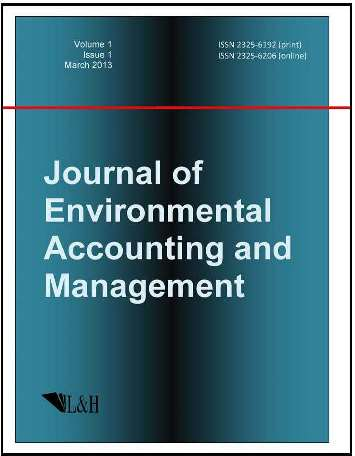ISSN:2325-6192 (print)
ISSN:2325-6206 (online)
Journal of Environmental Accounting and Management
António Mendes Lopes (editor), Jiazhong Zhang(editor)
António Mendes Lopes (editor)

University of Porto, Portugal

Email: aml@fe.up.pt

Jiazhong Zhang (editor)

School of Energy and Power Engineering, Xi'an Jiaotong University, Xi'an, Shaanxi Province 710049, China

Fax: +86 29 82668723 Email: jzzhang@mail.xjtu.edu.cn

Exact Solutions Involving two Jacobi Elliptic Functions for General Boussinesq Equation

Journal of Environmental Accounting and Management 10(1) (2022) 7--17 | DOI:10.5890/JEAM.2022.03.002

S. P. Joseph

Government Engineering College, Wayanad, Kerala, India

Abstract

In this paper, several new exact traveling wave solutions for a general Boussinesq equation are derived. Boussinesq equation is a fourth order nonlinear partial differential equation which is used to study dynamics of water waves in fluid dynamics and other physical phenomena such as the dynamics of thin inviscid layers, non-linear lattice waves and vibration in nonlinear strings. We derive all the exact solutions which are the linear combinations of square of two Jacobi elliptic functions and a constant term. Since the computations are much more involved, required solutions are obtained using computer algebra system.

References

1.   Biswas, A., Milovic, D., and Ranasinghe, A. (2009), Solitary waves of Boussinesq equation in a power law media, Commun. Nonlinear Sci. Numer. Simulat., 14, 3738-3742.
2.   Boussinesq, J. (1871), Theorie de lintumescence liquide appel ee onde solitaire ou de translation, se propageant dans un canal rectangulaire, C. R. Acad. Sci. Paris, 72, 755-759.
3.   Boussinesq, J. (1872), Theorie des ondes et des remous qui se propagent le long d un canal rectangulaire horizontal, en communiquant au liquide continu dans ce canal des vitesses sensiblement pareilles de la surface au fond, J. Math. Pures Appl., 17, 55-108.
4.   Ekici, M., Mirzazadeh, M., and Eslami, M. (2016), Solitons and other solutions to Boussinesq equation with power law nonlinearity and dual dispersion, Nonlinear Dyn., 84, 669-676.
5.   Fan, E. (2000), Extended tanh-function method, and its applications to nonlinear equations, Phys. lett. A, 277, 212-218.
6.   Hafez, M.G. (2016), New traveling wave solutions of the (1+1)-dimensional cubic nonlinear Schrodinger equation using novel (G'/G)-expansion method, Beni-Suef university journal of Basic and Applied Sciences, 5, 109-118.
7.   Hereman, W. and Nuseir, A. (1997), Symbolic methods to construct exact solutions of nonlinear partial differential equations, Math. Comput. Simul., 43, 13-27.
8.   Hirota, R. (2004), The Direct Method in Soliton Theory, Cambridge University Press, Cambridge.
9.   Joseph, S.P. and Gireesan, K.K. (2020), New exact solutions for general Boussinesq equation, Advances in Mathematics: Scientific Journal, 9(4), 2309-2315.
10.   Liu, S., Fu, Z., Liu, S., and Zhao, Q. (2001), Jacobi elliptic function expansion method and periodic wave solutions of nonlinear wave equations, Physics Letters A, 289(1-2), 69-74.
11.   Malfliet, W. and Hereman, W. (1996), The tanh-method part1. Exact solutions of nonlinear evolution and wave equations, Phys. Script, 54, 563-568.
12.   Olver, F.W.J., Lozier, D.W., Boisvert, R.F., and Clark, C.W. (2010), NIST Handbook of Mathematical Functions, Cambridge University Press, Cambridge.
13.   Oxley, T., Jeffrey, P., and Lemon, M. (2002), Policy Relevant Modelling: Relationships Between Water, Land Use, and Farmer Decision Processes, Integrated Assessment, 3(1), 30-49.
14.   Wang, M., Li, X., and Zhang, J. (2008), The (G'/G)-expansion method and travelling wave solutions of nonlinear evolution equations in mathematical physics, Phys. Lett. A, 372, 417-423.
15.   Wazwaz, A.M. (2016), Multiple soliton solutions and multiple complex soliton solutions for two distinct Boussinesq equations, Nonlinear Dyn., 85, 731-737.
16.   Wazwaz, A.M. (2008), New travelling wave solutions to the Boussinesq and the Klein-Gordon equations, Commun. Nonlinear Sci. Numer. Simul., 13, 889-901.
17.   Zayed, E.M.E. and Abdelaziz, M.A.M. (2011), Exact solutions of the nonlinear Schrodinger equation with variable coefficients using the generalized extended tanh-function method, the sin-cosine and the exp-function methods, Applied Mathematics and computation, 218, 2259-2268.